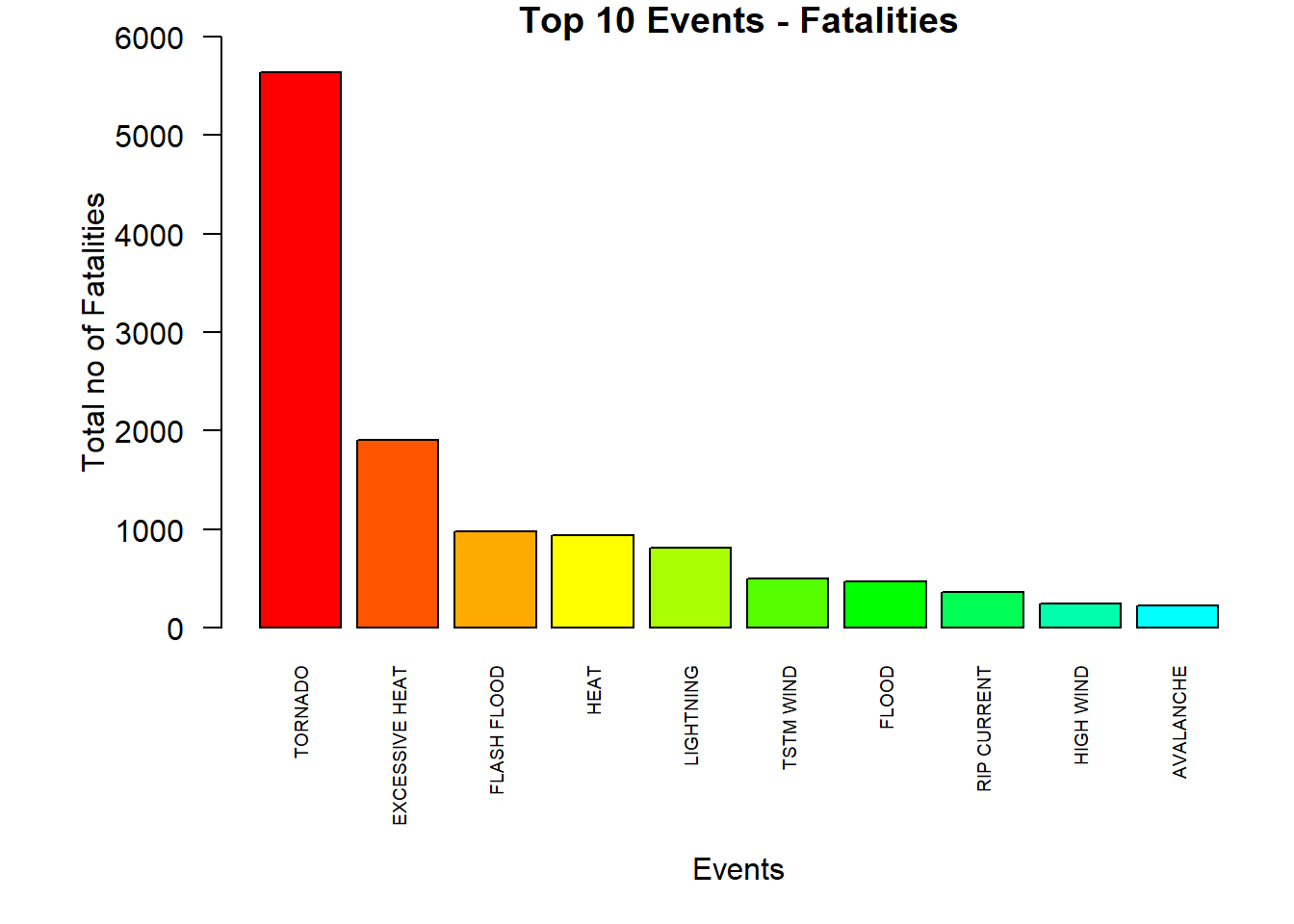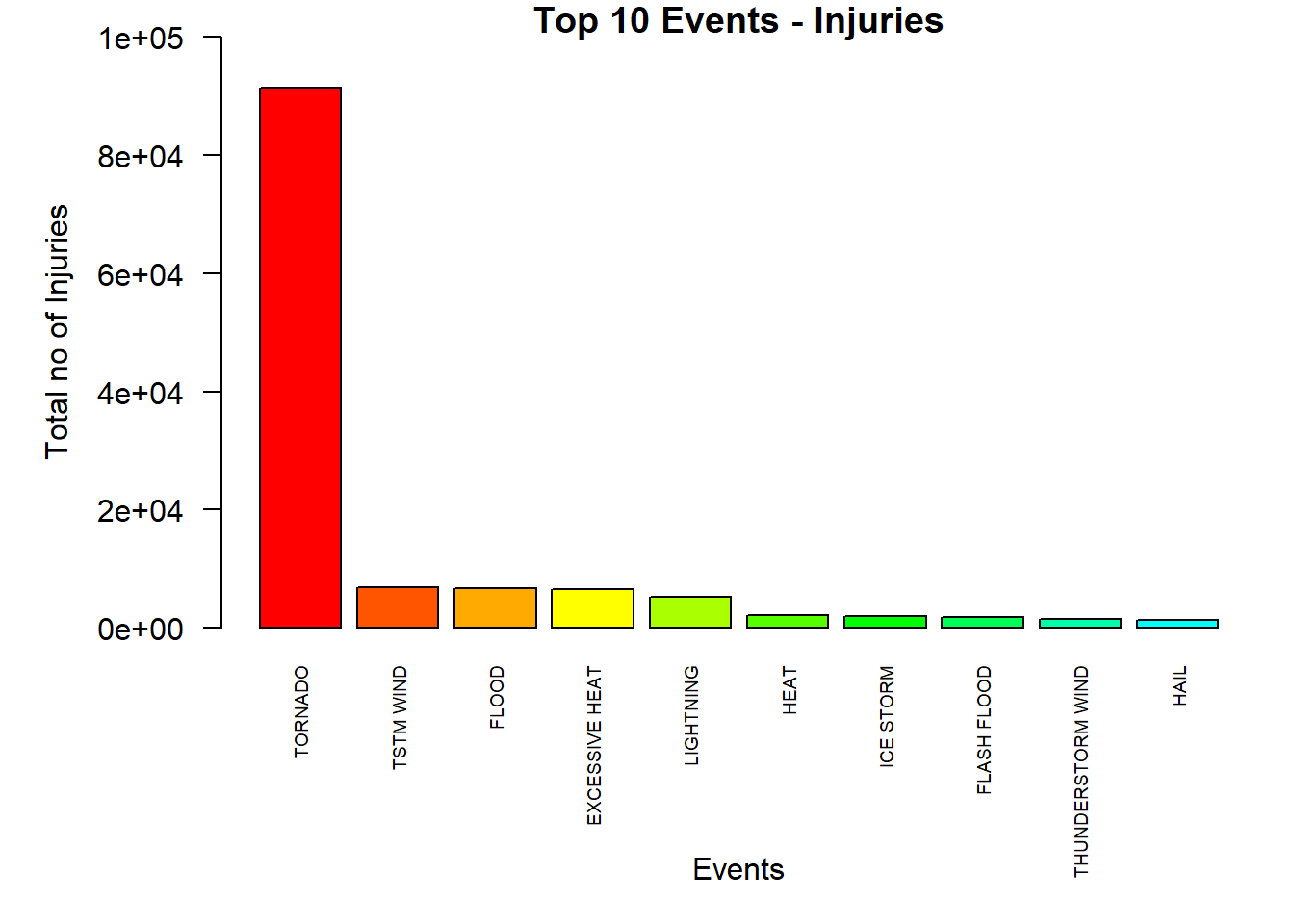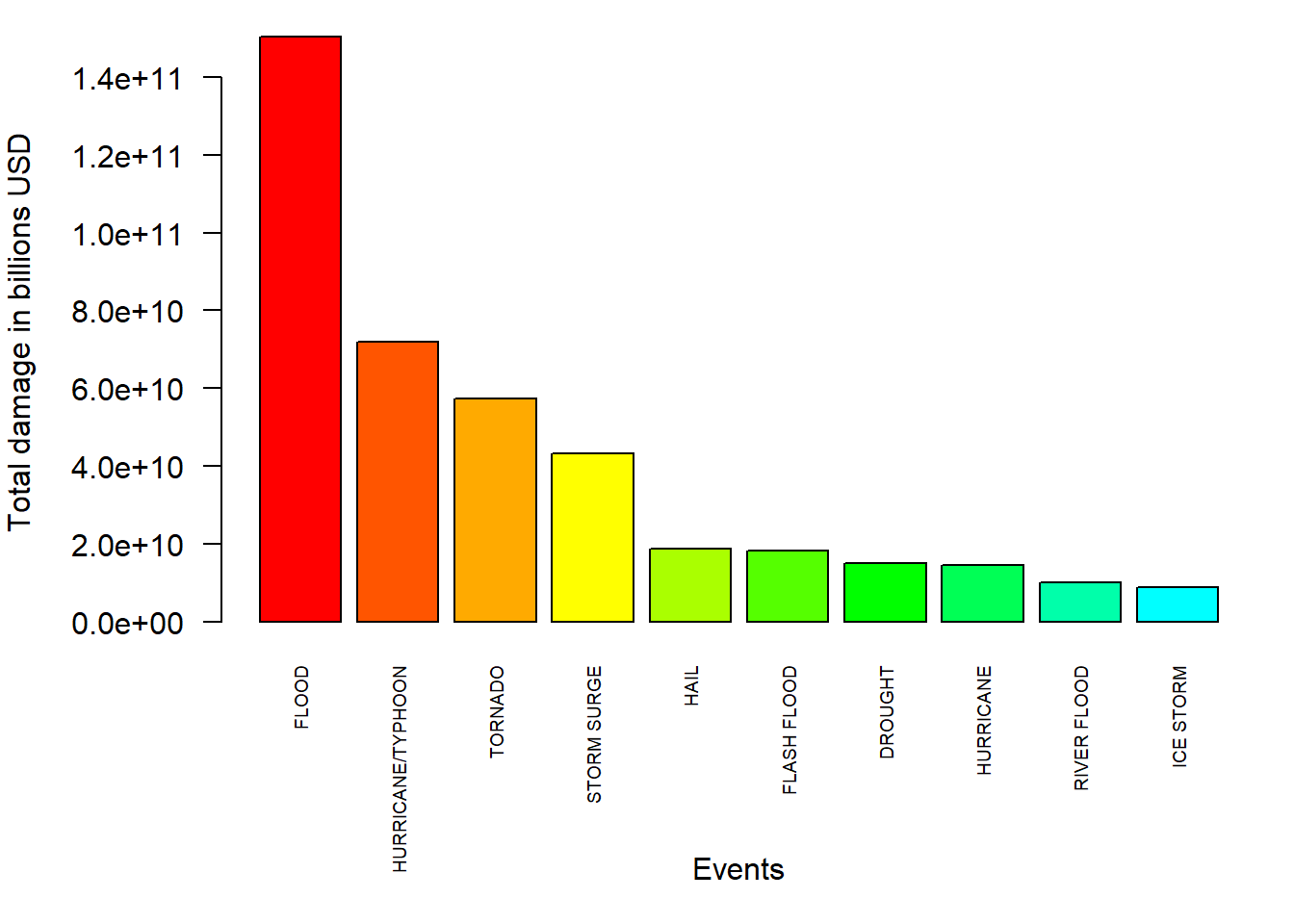## Introduction

The goal of this assignment is to analyze the NOAA Storm Database and answer the relevant questions about severe weather events. The database start in the year 1950 and end in November 2011.

This analysis addresses the following questions mentioned below:

• Across the United States, which types of events are most harmful with respect to population health?
• Across the United States, which types of events have the greatest economic consequences?

## Synopsis

We will obsereve that tornados have caused the maximum fatalities and injuries, and the floods has caused the highest value of property & crop damage.

library(ggplot2)

url <- "https://d396qusza40orc.cloudfront.net/repdata%2Fdata%2FStormData.csv.bz2"

data <- read.csv("data.csv",header = T,stringsAsFactors = F)

## Data Processing

• Taking out the variables which we need for this analysis.
data <- data[,c("STATE", "EVTYPE", "FATALITIES", "INJURIES", "PROPDMG", "PROPDMGEXP","CROPDMG", "CROPDMGEXP")]

head(data)
##   STATE  EVTYPE FATALITIES INJURIES PROPDMG PROPDMGEXP CROPDMG CROPDMGEXP
## 1    AL TORNADO          0       15    25.0          K       0
## 2    AL TORNADO          0        0     2.5          K       0
## 3    AL TORNADO          0        2    25.0          K       0
## 4    AL TORNADO          0        2     2.5          K       0
## 5    AL TORNADO          0        2     2.5          K       0
## 6    AL TORNADO          0        6     2.5          K       0

## Results

• Fatalities

We are looking here at the type of event which caused the maximum number of fatalities throughout the years:

fatalities <- aggregate(FATALITIES~EVTYPE, data, sum)
fatalities <- fatalities[order(-fatalities$FATALITIES),] par(mar=c(8, 6, 1, 1)) barplot (height = fatalities$FATALITIES[1:10], names.arg = fatalities$EVTYPE[1:10], las = 2, cex.names= 0.6, col = rainbow (10, start=0, end=0.5),ylim = c(0,6000),ylab = "Total no of Fatalities") mtext(side = 1, text = "Events", line = 6) title("Top 10 Events - Fatalities")• Injuries We are looking here at the type of event which caused the maximum number of Injuries throughout the years: Injuries <- aggregate(INJURIES ~ EVTYPE, data, sum) Injuries <- Injuries[order(-Injuries$INJURIES),]
par(mar=c(8, 6, 1, 1))
barplot (height = Injuries$INJURIES[1:10], names.arg = Injuries$EVTYPE[1:10], las = 2, cex.names= 0.6,
col = rainbow (10, start=0, end=0.5),ylim = c(0,100000))

mtext(side = 1, text = "Events", line = 6)
mtext(side = 2, text = "Total no of Injuries", line = 4)

title("Top 10 Events - Injuries")• Property & Crops

The United States, which types of events have the greatest economic consequences

character <- c("", "+", "-", "?", 0:9, "h", "H", "k", "K", "m", "M", "b", "B")
factor <- c(rep(0,4), 0:9, 2, 2, 3, 3, 6, 6, 9, 9)

exponent <- data.frame(character, factor)

data$prop_damage <- data$PROPDMG*10^exponent[match(data$PROPDMGEXP,exponent$character),2]
data$crop_damage <- data$CROPDMG*10^exponent[match(data$CROPDMGEXP,exponent$character),2]

data$total_damage <- data$prop_damage + data$crop_damage final <- aggregate(total_damage ~ EVTYPE, data, sum) final <- final[order(-final$total_damage),]

par(mar=c(8, 6, 1, 1))
barplot (height = final$total_damage[1:10], names.arg = final$EVTYPE[1:10], las = 2, cex.names= 0.6,
col = rainbow (10, start=0, end=0.5))

mtext(side = 1, text = "Events", line = 6)
mtext(side = 2, text = "Total damage in billions USD", line = 5)## Conclusion

The plots show that tornados have caused the maximum fatalities and injuries, and the floods has caused the highest value of property & crop damage.